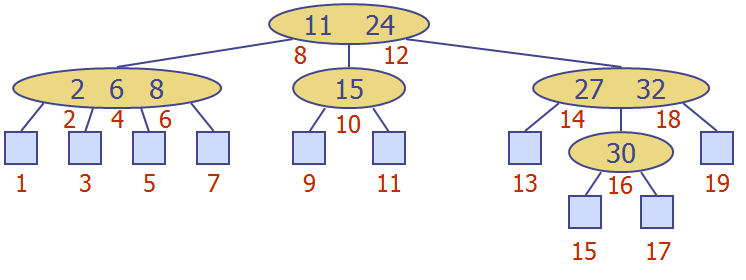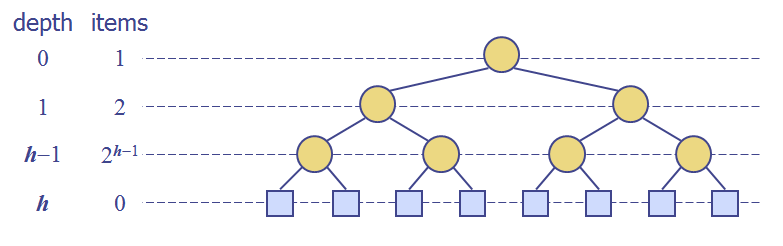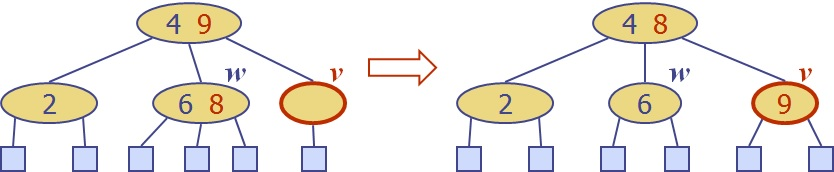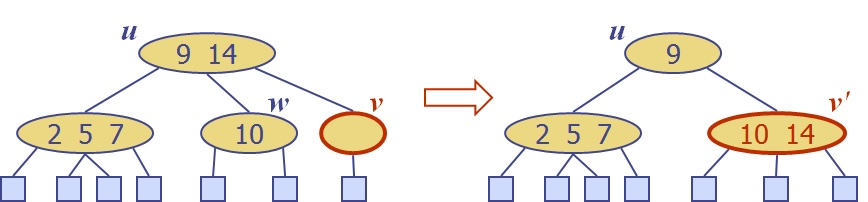# 磨刀不误砍柴工 系列之 二 : Bounded-Depth Search Trees

Bounded-Depth Search Trees includes: (2,4) Tree, Red-Black Tree

To under Red-Black tree more easily, we could learn (2,4) Tree at first. These two trees can be converted to each other.

Bounded-Depth Tree, or Depth-Bounded Tree, can be used to implement an ordered dictionary with $$O(logn)$$ search and update times.## (2,4) Tree

Size Property: Every nopde has at most four children.

Depth Property: All the external nodes have the same depth.

A (2,4) tree storing $$n$$ items has height $$O(logn)$$.

Searching in a (2,4) tree with $$n$$ items taks $$O(logn)$$ time.Overflow: If a node $$v$$ was previously a 4-node, then it may become a 5-node after the insertion. Overflow must be resolved in order to restore the properties of a (2,4) tree. The point is: take $$k3$$ out of this node and insert it into the parent node $$u$$. The overflow may propagate to the parent node $$u$$. A split operation affects takes $$O(1)$$ time; while overflow propagates, the total time to perform an insertion in a (2,4) tree is $$O(logn)$$.Underflow: If $$v$$ was previously a 2-node, then it becomes a 1-node with no items after the removal. To remedy an underflow, we have two operations: transer or fusion.
we perform a transfer operation, in which we move a child of $$w$$ ($$v's$$ sibling) to $$v$$;or we perform a fusion operation, in which we merge $$v$$ with a sibling, creating a new node $$v'$$, and move a key from the parent $$u$$ of $$v$$ to $$v'$$.Whether we will take transfer or fusion operation depends on, the immediate sibling of $$v$$ is 3+-nodes or 2-nodes.

## Red-Black Tree

A red-black tree is a BST that uses a kind of “perudo-depth” to achieve balance using the approach of a depth-bounded search tree.
In particular, it’s a BST with nodes colored red and black in a way that stisfies the following properties:

Root Property: The root is black.

External Property: Every external node is black.

Internal Property: The children of a red node are black.

Depth Property: All the external nodes have the same black depth, which is defined as the number of black ancestors minus one.Red-black tree associated with the (2,4) tree

Performing the update operations in a red-black tree is similar to that of a BST, except that we must additionally restore the color properties.

new node, let it be z, inserted is initially proceeds as in a BST and colored red(if z is not root). Color the two external-node children of z black. This action preserves the root, external and depth properties of T, but it may violate the internal property. Then, we have to do recoloring and rotating if necessary.

The insertion of a key-element item in a red-black tree storing n items can be done in O(logn) time and requires at most O(logn) recolorings and one trinode restructuring (a restruecture operation)

 Tweet